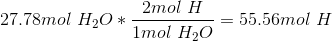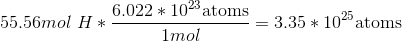# High School Chemistry : Using Avogadro's Number

## Example Questions

← Previous 1

### Example Question #11 : Units

How many atoms are in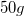of calcium?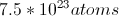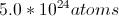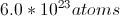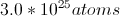Explanation:

In order to determine how many atoms are in this sample, we need to convert this sample into moles. Calcium has a molar mass of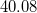grams per mole.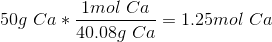Avogadro's number tells us that there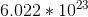atoms in one mole of any element. We can use this conversion to find the total number of atoms in the sample.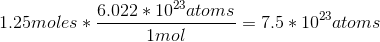### Example Question #2 : Using Avogadro's Number

How many atoms are there in 47.5g of boron?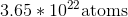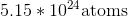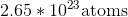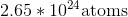Explanation:

To do this problem we have to first convert grams to moles, then moles to atoms using Avogadro's number: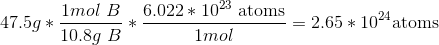### Example Question #3 : Using Avogadro's Number

How many moles of carbon are in a sample of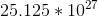atoms?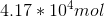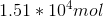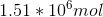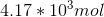Explanation:

To solve, we need to convert atoms to moles using Avogadro's number: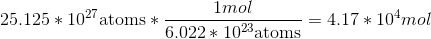### Example Question #2 : Using Avogadro's Number

How many atoms of sodium are in three moles of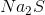?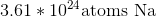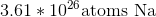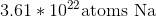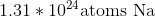Explanation:

To answer this question, we have to find the number of moles of sodium in this compound. Note that there are two sodium atoms per molecule. Then, multiply the total moles of sodium by Avogadro's number.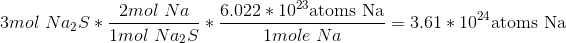### Example Question #1 : Using Avogadro's Number

You have a neutral balloon. If you were to add 21,000 electrons to it, what would its net charge be?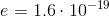= charge of one electron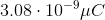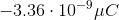None of the other answers is correct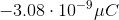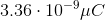Explanation:

The elemental charge is the magnitude of charge, in Coulombs, that each electron or proton has. Because electrons have a negative charge, don't forget to add a negative sign into the equation.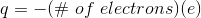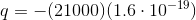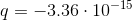When you convert the answer to microcoulombs, the answer is: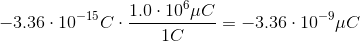### Example Question #1 : Using Avogadro's Number

What is the mass of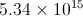particles of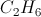?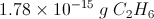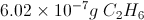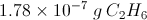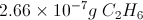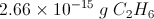Explanation: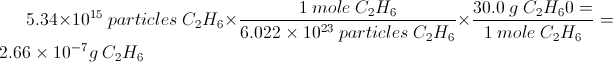### Example Question #1 : Stoichiometry

How many atoms are in 1 mole of H2?

1.2044 * 1024

3.626 * 1047

6.022 * 1046

6.022 * 1023

1.2044 * 1024

Explanation:

This question requires an understanding of what avogadro's number actually represents.  Avogadro's number, 6.022 * 1023 is the number of things in one mole.  The question indicates that there is 1 mole of H2.  Thus there are 6.022 * 1023 molecules of H2.  However the question is asking for the amount of atoms in 1 mole of H2.  Thus we must consider the makeup of an H2 molecule, where we see that it is a diatomic molecule.  Thus we must multiply 6.022 * 1023 by 2 to calculate the number of individual atoms present in 1 mole of H2.  We find our answer to be 1.2044 * 1024.

### Example Question #4 : Using Avogadro's Number

A chemist has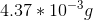of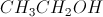.

How many molecules ofdoes she have?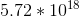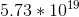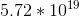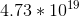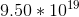Explanation: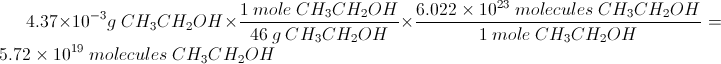### Example Question #2 : Stoichiometry

How many hydrogen atoms are in 46.3 g of ethanol,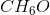?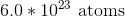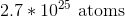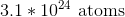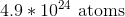Explanation:

To determine the number of hydrogen atoms, divide the mass of ethanol by its molar mass to get moles of ethanol.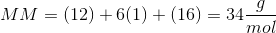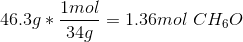Multiply this by six atoms of hydrogen per molecule of ethanol and by Avogadro's number to get the number of hydrogen atoms.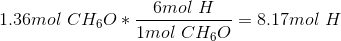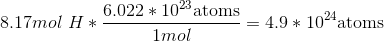### Example Question #1 : Using Avogadro's Number

How many hydrogen atoms are present in 500 mL of water at room temperature?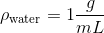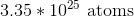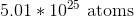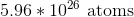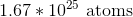Explanation:

Use the density of water, the molar mass of water, and Avogadro's number to calculate the number of molecules of water.

We have 500mL of water. Use the density to convert this to grams; then use the molar mass of water to convert this to moles.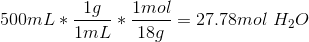There are two moles of hydrogen atoms per one mole of water.